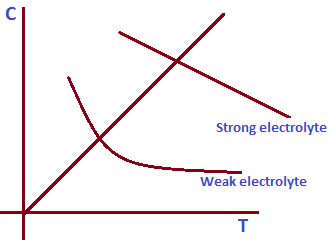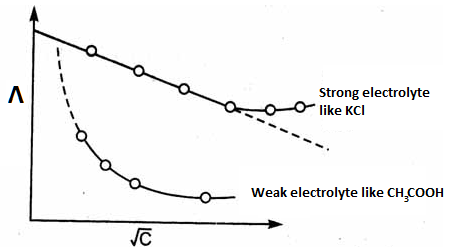# Variation of Conductance

The conductance of an electrolytic solution depends upon the number of ions and mobility of ions in it. The solution which is affected by different factors:

1. Effect of temperature: In case of strong electrolysis, the conductance increases with the increase in temperature due to mobility of ions.

But, in case of weak electrolyte, the conductance increase with the increase in electrolysis due to increase in both number of ions and mobility of ions. The below graph shows the relation between concentration and temperature for both type of electrolysis.Concentration vs temperature graph

2. Effect of Concentration: In case of strong electrolyte, specific conductance  decreases with the increase in dilution due to increase in number of ions per unit volume. But, equivalent conductance and molar conductance increases with the increase in dilution due to increase in mobility of ions.

In case of weak electrolyte, specific conductance increase with the increase in dilution due to the increment in both number of ions and mobility of ions.

When we plot the graph of variation of equivalent conductance against square root of concentration, we get curved graph for weak electrolyte and a straight line for strong electrolyte given as below.Variation of equivalent conductance with concentration

Do you like this article ? If yes then like otherwise dislike : 5